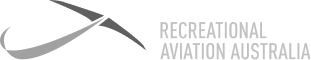# Definition: Above Ground Level (AGL)

The distance measured between the aircraft and any part of the ground. A common use for AGL is for parachuting, where the distance to the ground is more important than the distance above mean sea level. Normally, when on the ground, the altimeter is set to 0 feet, which in most cases will not be the current pressure setting that would normally be set on the altimeter to give AMSL.### Above Ground Level (AGL)

The distance measured between the aircraft and any part of the ground. A common use for AGL is for parachuting, where the distance to the ground is more important than the distance above mean sea level. Normally, when on the ground, the altimeter is set to 0 feet, which in most cases will not be the current pressure setting that would normally be set on the altimeter to give AMSL.

7 Day

FREE

Trial